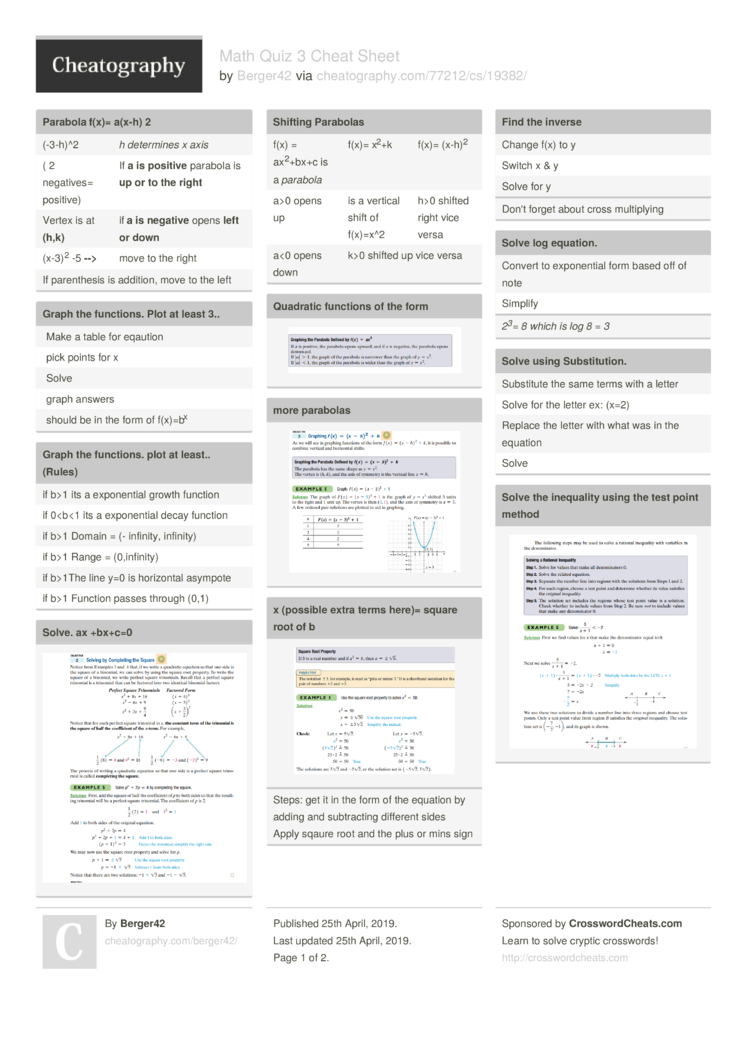Show Menu

# Math Quiz 3 Cheat Sheet by Berger42

### Parabola f(x)= a(x-h) 2

 (-3-h)^2 h determines x axis ( 2 negatives= positive) If a is positive parabola is up or to the right Vertex is at (h,k) if a is negative opens left or down (x-3)2 -5 --> move to the right If parent­hesis is addition, move to the left

### Graph the functions. Plot at least 3..

 Make a table for eqaution pick points for x Solve graph answers should be in the form of f(x)=bx

### Graph the functions. plot at least.. (Rules)

 if b>1 its a expone­ntial growth function if 01 Domain = (- infinity, infinity) if b>1 Range = (0,inf­inity) if b>1The line y=0 is horizontal asympote if b>1 Function passes through (0,1)

### Solve. ax +bx+c=0### Shifting Parabolas

 f(x) = ax2+bx+c is a parabola f(x)= x2+k f(x)= (x-h)2 a>0 opens up is a vertical shift of f(x)=x^2 h>0 shifted right vice versa a<0 opens down k>0 shifted up vice versa

### Quadratic functions of the form### more parabolas### x (possible extra terms here)= square root of bSteps: get it in the form of the equation by adding and subtra­cting different sides
Apply sqaure root and the plus or mins sign

### Find the inverse

 Change f(x) to y Switch x & y Solve for y
Don't forget about cross multip­lying

### Solve log equation.

 Convert to expone­ntial form based off of note Simplify
23= 8 which is log 8 = 3

### Solve using Substi­tution.

 Substitute the same terms with a letter Solve for the letter ex: (x=2) Replace the letter with what was in the equation Solve

### Solve the inequality using the test point method### Logarithms

 log 1=1 Only solve multiple logs if they have the same base log bx =x log (xy) = log (x) + log (y) blog sub bx =x log 1=0 log b=1
power is what it's equal to
base is the sub

### f and g functions

 replace f and g perform operation in middle Horizontal line test (g ∘ f )(x) = g(f(x)) intersects more than once, not a function

### Graphing inverse

 Look at > or < sign, determines what part of parabolas are the answer Find the inverse plot parabola from solved equation "­y=..." Make a table of points

### Solve the equation b = c

 Get bases same by putting a power or square root or fraction Cross out bases Exponents become base Solve equation
Sometimes doesnt look like example, general it has 2 numbers raised to a power with an equal sign between

### Quadratic Formula### Solving a Polynomial Inequality

 Think of it as a Quadratic equation (< OR > as a = sign) Solve equation Plot answers Pick numbers from each A,B,C if equation is tue part of solution if false not part of solution find and write out solution set

### Solving a Polynomial Inequality EX### Vertex Formula### Didn't go over

 9.3-­9.6

## Download the Math Quiz 3 Cheat Sheet2 Pages
//media.cheatography.com/storage/thumb/berger42_math-quiz-3.750.jpg

PDF (recommended)

Alternative Downloads

## Comments

No comments yet. Add yours below!

## Add a Comment

Your Comment

Please enter your name.

Please enter your email address

Please enter your Comment.

## Related Cheat Sheets

Linear Algebra Cheat Sheet
Algebra II Final
Laws of Boolean Algebra Cheat Sheet

## More Cheat Sheets by Berger42

Spanish finals Cheat Sheet
Math M03 quiz 1 Cheat Sheet
Math M03 Quiz #2 Cheat Sheet